# Reciprocals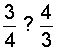Example 1: What is the relationship between three-fourths and four-thirds?

Analysis: Let's try finding three-fourths of four-thirds. The word OF means "times", which indicates that we need to multiply.

Solution: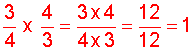Answer: The product of three-fourths and four-thirds is 1.

This product is no coincidence. Three-fourths and four-thirds are reciprocals.

Definition:   Two numbers whose product is 1 are called reciprocals of each other.

Reciprocals come in pairs. We can find the reciprocal of a nonzero number in fractional form by inverting it (that is, by switching positions of the numerator and denominator).

### Watch This Fraction in Action!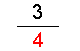Let's look at some more examples of reciprocals.

Example 2: The following pairs of numbers are reciprocals.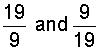Note that the last fraction listed above was an improper fraction. To find the reciprocal of a mixed number, you must convert it to an improper fraction first.

Example 3: What is the reciprocal of 3 and 1/2?

Solution Step 1: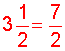Solution Step 2:Example 4: What is the reciprocal of 5 and 7/8?

Solution Step 1: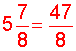Solution Step 2: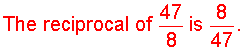Summary: Two numbers whose product is 1 are called reciprocals of each other.

### Exercises

Directions: Find the reciprocal of each number below. Click once in an ANSWER BOX and type in your answer; then click ENTER. After you click ENTER, a message will appear in the RESULTS BOX to indicate whether your answer is correct or incorrect. To start over, click CLEAR.

Note: To write the fraction three-fourths, enter 3/4 into the form.

 1.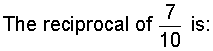ANSWER BOX:   RESULTS BOX:
 2.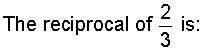ANSWER BOX:   RESULTS BOX:
 3.ANSWER BOX:   RESULTS BOX:
 4.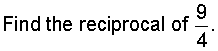ANSWER BOX:   RESULTS BOX:
 5.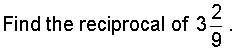ANSWER BOX:     RESULTS BOX:
 Lessons on Multiplying and Dividing Fractions and Mixed Numbers 1. Multiplying Fractions 2. Multiplying Fractions by Cancelling Common Factors 3. Multiplying Mixed Numbers 4. Reciprocals 5. Dividing Fractions 6. Dividing Mixed Numbers 6. Solving Word Problems 7. Practice Exercises 8. Challenge Exercises 9. Solutions